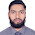Definition and types of yarn count

What is yarn count | Different types of count

Yarn count
The yarn count is a numerical expression which defines its fineness or coarseness. It also expresses weather the yarn is thick or thin. A definition is given by the textile institute – “Count is a number which indicates the mass per unit length or the length per unit mass of yarn.”

Types of yarn count

- Indirect system- English, Metric, Worsted.
- Direct system- Tex, Denier, Lbs/Spindle.

Indirect count
The count of yarn expresses the number of length units in one weight unit. Thus higher the count, finer the yarn. The system is generally used for cotton, worsted, linen (wet spun) etc.
w x L
Indirect count: N= ………………
W X l
Where, W = The weight of the sample.
w = The unit weight of the system.
L = The length of the sample.
l = The unit length of the sample.

Direct count
The count of yarn expresses the no. of weight units in one length unit. Thus higher the count, coarser the yarn. The system is generally used for synthetic fibre, jute, silk etc.
W X l
Direct count: N = …………
w X L
Where,
N = The yarn number or, count.
W = The weight of the sample.
w = The unit weight of the sample.
L = The length of the sample.
l = The unit length of the sample.

In Details:
English system: It is defined as the number of hanks (840 yds) per pound. count.
Length (yds)     1 pound
English count N =……………… X ………………….....
840 yds             Weight (Pound)
Metric system: It is defined as the number of hank (1000m) per kg.
Length (m)           1 kg
Metric count =……………X ………………...........
1000m            Weight (kg)
Worsted system: It is defined as the number of hanks (540yds) per pound.
Length (yds)     1 Pound
Worsted count =………………X ………………….....
560 (yds)          Weight (Pound)
Tex system or Lea count: It is defined as the weight in grams of 1000 m.
Weight (gm)     1000m
Tex count =……………X  …………….......
1 gm                 Length (m)
Denier: The number or, count in the denier system is the weight in grams of 9000 m.
Weight (gm)       9000m
Denier count =……………X…………..........
1 gm                   Length
Pounds per spindle (Jute system): Count in the pound per spindle system is the weight in pounds of 14400 yards of yarn.
Weight (Pound)   14400 (yds)
Pound per spindle =………………. X……………........
1 Pound                Length (yds)

Previous Post
Next Post

Md. Abu Sayed

He is a Textile Engineer and like writing on Apparel

Related Posts

1.It is a very essential topic....

2.You have a nice blog with informative articles. I was searching the key ward "yarn count" and got your blog. It helped me the most. Thanks.

3.thanks for your sharing. I learned a lot from your post. And how much do you know about yarn count's advantages? And disadvantages?

4.5.Nice it's help ful